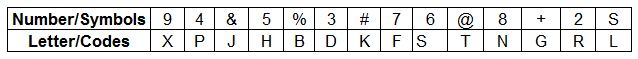### IBPS Clerk 15-Dec-2012 Question 186

Instructions

In each question below is given a group of numbers/symbols followed by five combinations of letter codes numbered a: , b:.c:. d: and e:. You have to find out which of the combinations correctly represents the group of numbers/symbols based on the following coding system and the conditions and mark the number of that combination as your answer.Conditions :
(i) If the first element is a symbol and the last element is a number, then the codes for both are to be interchanged.
(ii) If both the first and last elements are symbols, then the last element is to be coded as the code lor the first element.
(iii) If the group of elements contains only one symbol, then that symbols is to be coded as A.

Question 186

# 28%956

Solution

The element, 28%956 , contains only 1 symbol.

Thus, applying rule (iii), the code for '%' - 'A', and rest of the codes will remain the same.

=> 28%956 - RNAXHS